Help | Chaos Webbook | ProjectsNext lecture ]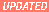23 Nov 1998

DIFFUSION, TRANSPORT AND NON-EQUILIBRIUM STATISTICAL MECHANICS

Fall semester 1998
Predrag Cvitanovic´

Current schedule: http://www.nbi.dk/~predrag/NBIcourses/Fysik711B-98-sched.html
For people following the course, check the e-mail list.

Dorfman problem sets

Lecture 1           13:15 - 15:00 Thursday, 3 Sep 98 in Aud. C - NBI Blegdamsvej
Boltzmann equation
We start with the classic - a heuristic derivation of the 1872 Boltzmann equation for density of a dilute gas.
Reading: chapters 1-3, section 3.1 of Dorfman's notes.
Other literature of interest: Thompson sect. 1.4, Harris chapter 3.

Problem set session            10:15 - 12:00 Friday, 4 Sep 98 in Aud. B - NBI Blegdamsvej
Discussion led by Paolo Muratore-Ginanneschi - derivation of the Boltzmann equation kernel. Binary collision integrals are worked out in detail in ref. .
Problem: Dorfman's 1.1 Ehrenfest wind-tree model
Due Fri 11 Sep 98

Lecture 2           13:15 - 15:00 Thursday, 10 Sep 98
Boltzmann H-theorem; Kac's ring model
H - theorem states that even though the microscopics dynamics is reversible, the gas tends irreversibly toward the equilibrium. How can it be? hang on for the rest of the course. Kac's ring is a simple model illuminating aspects of Stosszahlansatz; macroscopic irreversibility from microscopic reversibility and a Poincare recurrence.
Problem: Present a derivation of the Maxwell-Boltzmann distribution for a gas in thermal equilibrium (you can follow your favorite statistical mechanics textbook). Check that this is a (the?) solution of the constant H(t) condition.

Lecture 3           13:15 - 15:00 Thursday, 17 Sep 98
Tagged particle diffusion
An illustration of the Chapman-Enskog method for solving the Boltzmann equation; we derive the diffusion equation and compute the diffusion coefficient.

Problem set session            10:15 - 12:00 Friday, 18 Sep 98 in Aud. B - NBI Blegdamsvej
Discussion led by Paolo
Problems: Maxwell-Boltzmann distribution, Dorfman problem 1.2 - Lorentz gas (optional)
Due Fri 18 Sep 98

Lecture 4           13:15 - 15:00 Thursday, 24 Sep 98
Liouville's equations
We derive Liouville's equation for the full N-particle phase-space, and sketch the BBGKY hierarchy of equations derived from it, with the Boltzmann equation as the lowest order approximation.
Reading: chapters 4, 5, sects. 4.1-4.2
Chapter 4 Local dynamics, quantitative, sects 4.1 - 4.1.1
Chapter 6 Transporting densities, sects 6.1 - 6.2
Other literature of interest: Thompson, Appendix A for Liouville theorem.

Problems:
4.1 Go through the steps of the tagged particle diffusion omitted in the lecture (don't count on Paolo doing it for you -  be prepared to just do it, on the blackboard)
Due Fri 25 Sep 98

Problems (next week):
5.1 Derive the first BBGKY hierarchy equation, Dorfman (4.7).
5.2 (K. Huang's 4.6):
A room of volume 3 \times 3 \times 3 cubic meters is under standard conditions (atmospheric pressure and 300 K).
a) Estimate the probability that at any instant of time a 1-cc volume anywhere within this room becomes totally devoid of air because of spontaneous statistical fluctuations.
b) Estimate the same for a 1-\Aangstrom^3 volume.
5.3 (K. Huang's 4.7):
Suppose the the situation referred to in Problem 4.6a has occured. Describe qualitatively the behavior of the distribution function thereafter. Estimate the time it takes for such a situation to occur again, under the assumption that molecular collisions are such that the time sequence of the state of the system is a random sequence of states.
Due Fri 2 Oct 98

Lecture 5           13:15 - 15:00 Thursday, 1 Oct 98
Poincare recurrence theorem, Boltzmann hypothesis, Gibbs
A pasta lover's derivation of the Poincare recurrence theorem - you cannot wind an infinite-length spaghetti through a finite volume.  Dorfman notes then traverse territory certainly worth reading and understanding, but at this level  it is preaching to the converted and  probably best skipped in the lecture. I leave it as the reading assignement: Boltzmann's hypothesis equates microcanonical ensemble average with the infinite time average. Birkhoff proved that the time average exists for almost every initial condition.  Gibbs made a stronger, mixing assumption to describe the approach to the equilibrium. With some luck, we go beyond all these words and start getting our teeth into Green-Kubo formulas.
Reading: Dorfman chapter 5, sect. 5.1 .
Dorfman chapter 6, chapter 7, sects. 6.1-2, 7.1-3.

Problems: Dorfman sect 5.1 and problem 2.2 - the Ehrenfest urn model.
Dorfman 3.1.
Solution 2.2: in ref. , perhaps handed out in the problems session.
Solutions: Dorfman 3.1, 3.2, 4.1 .
Due Fri 9 Oct 98

Lecture 6          13:15 - 15:00 Thursday, 8 Oct 98
The Green-Kubo formulas for diffusion
Linear response theory  relates the electrical conductivity of a system of charged particles to the  time correlation function. We also recast the Liouville equation in a more general setting - in terms of operators that move the whole phase space around, and try to make sense of them. We then go through the standard derivation of the the Green-Kubo formulas for the diffusion coefficient in terms of the time velocity-velocity correlation function.
Reading: Dorfman chapter 8, sect. 8.1., 8.3
Chaos Webbook Chapter 5 - Transporting densities
Problems: 1-2, 1-5, 1-6, 1-7 and 1-8 from ref. , handed out in the lecture.
Due Fri 23 oct 98

Midterm take-home exam: compute the diffusion constant for the 2 or the 3-dimensional Lorentz gas.

kartofler!

Midterm take-home exam solution: The diffusion constant for the Lorentz gas is
D = 3v/(16 a n)    in 2 dimensions
D = v/(3 \pi a^2 n)    in 3 dimensions

where v=velocity, a=radius of the scatterers, and n=density of scattereres. Paolo will distribute copies of his calculation to the class. A nice discussion can be found in chapter 11 of ref. , Difusion of a light gas in a heavy gas''.

Lecture 7          13:15 - 15:00 Thursday, 22  oct 98
Qualitative dynamics
We now embark upon the modern'' track of the course: We will develop an exact theory of deterministic transport, without any statistical assumptions about collisions. We start by training on a simple gas'' - a point particle bouncing of 3 disks in a plane.
Qualitative dynamics of simple stretching and mixing flows is used to introduce Smale horseshoes and symbolic dynamics. One learns how to count and describe itineraries. The topological dynamics is incoded by means of transition matrices/Markov graphs.
Reading: Chaos Webbook Chapter 1 - Overture. Light bedside reading to give you sense for the grand scheme of things chaotic. Our path will be narrow - to statistical mechanics applications
Chaos Webbook Chapter 2 - Qualitative dynamics. Somewhat tedious but necessary set of stretching exercises to develop intution about chaotic flows.
Chaos Webbook chapter 1 exercises 1.1, 1.3 .
Problems: Chaos Webbook chapter 2 exercises 2.1, 2.2, 2.4 .
Due Fri 30 oct 98

Lecture 8          13:15 - 15:00 Thursday,  29 0ct 98
Learning how to count
The topological dynamics is incoded by means of transition matrices/Markov graphs, and while computing the topological entropy we encounter our first zeta function.
Reading: Chaos Webbook Chapter 3 - Counting.
Problems: Chaos Webbook chapter 3 exercises 3.1, 3.3, 3.9 optional: exercises 3.7, 3.15, 3.16, 3.17
Due Fri 6 Nov 98

Lecture 9           13:15 - 15:00 Thursday,  5 nov 98
Dynamics, local and global
We review the basic concepts of local dynamics required to move beyond mere counting and assign relative weights to topologically distinct parts of the phase space. Averages of observables in chaotic dynamics are related to the spectra of evolution operators.
Chapter 4 - Local dynamics, quantitative. Basic concepts of local dynamics required to move beyond mere counting and assign relative weights to topologically distinct parts of the phase space.
Chapter 6 - Transporting densities. A first attempt to move whole phase space around - we introduce fancy operators that we will actually not use in practice, discuss natural measure. Merits a quick read.
Chapter 7 - Global dynamics. The the core of the subject: we discuss the necessity of studying the averages of observables in chaotic dynamics, and cast the formulas for averages in a multiplicative form that motivates the introduction of evolution operators.
Problems: Chaos Webbook exercises 5.4, 5.9, 6.2, 7.1 - optional: Henon map exercises 6.3, 5.24.
Due Fri 13 Nov Dec 98

Lecture 10          13:15 - 15:00 Thursday,  12 Nov 98
Trace formulas, spectral determinants
This is the core chapter: If there is one idea that one should learn about chaotic dynamics, it happens in this chapter, and it is this: the spectrum of (global) eigenvalues is dual to the spectrum of (local) periodic orbits. Here one extracts the spectrum of evolution operators from the associated trace formulas, spectral determinants, dynamical zeta functions.
Reading: Chapter 8 - Traces and determinants
Problems: Chaos Webbook exercises 6.3, 8.1, 10.6; optional 6.4, 10.2.
Due Fri 20 Nov 98.

Lecture 11           13:15 - 15:00 Thursday,  19  Nov 98
Cycle expansions
In last two lectures we have derived a plethora of periodic orbit trace formulas, spectral determinants and zeta functions. Now we learn how to expanded these as cycle expansions, series ordered by increasing topological cycle length, and evaluate average quantites like escape rates. These formulas are exact, and, for nice flows, highly convergent. The pleasant surprise is that the terms in such expansions fall off exponentially or even faster, so that a handful of shortest orbits suffices for rather accurate estimates of asymptotic averages.
Chapter 9 - Cycle expansions
Chapter 10 - Getting used to cycles
Problems: Chaos Webbook exercises 10.3, 10.6, 9.16 (9.16 requires some easy computer programing; if you really understand it you will get some feeling fow strange strange'' attractors really are)
Due Fri 27 Nov 98.

3/4-term take-home exam (10% of the grade): Do complete Chaos Webbook exercises 10.1 and 9.2 - escape rate for a 1-dimensional repeller.   A heavy handed hint: check the solutions to the problems. Deliver the solution (including discussion of various steps in the problems, printouts of your programs and their numerical results to Paolo
no later than Thur 3 dec 98.

Lecture 12          13:15 - 15:00 Thursday, 26 Nov 98
Non-equilibrium transport - Carl P. Dettmann
We are now ready to return to the problems that confronted us for the first half of the course: The computation of transport coefficients, and the nature of irreversibility in statistical mechanics. We look at some alternatives to Green-Kubo, and recycle the periodic Lorentz gas.
(optional): Morriss and Dettmann article on thermostats; We will touch on the escape rate formalism, covered in great detail in Gaspard's book, chapter 6; finally, there are hand-out notes by Evans and Morriss on computer simulation methods.
Problems

Due Fri Dec 4.

Lecture 13          13:15 - 15:00 Thursday, 3  Dec 98
Diffusion confusion
We compute diffusion constant for a simple 1-d model of deterministic diffusion.
Reading: Chaos Webbook Diffusion chapter 14.1, 14.2 (not 14.3)
Problems: Chaos Webbook exercises 14.1, optional: 14.2
Due Fri Dec 11.

Lecture 14           13:15 - 15:00 Thursday,  10  Dec 98
??Title
Term papers will take 2 weeks from start to finish. I am still formulating the projects and am open to suggestions.

Lecture  17 (the last of the course)           13:15 - 15:00 Thursday, 17 Dec 98
What's wrong with this course?
??summary.

Term papers         due no later than 16:00 Wed 27 Jan 1999 - Dorte Glass office

## References

1
G.E. Uhlenbeck, G.W. Ford and E.W. Montroll, Lectures in Statistical Mechanics (Amer. Math. Soc., Providence R.I., 1963).
2
S. Harris, An introduction to the Theory of the Boltzmann Equation (Holt, Rinehart and Winston, New York, 1971).
3
M. Kac, Random walk and the theory of Brownian motion'', (1946), reprinted in ref. .
4
N. Wax, ed., Selected Papers on Noise and Stochastic Processes (Dover, New York 1954).
6
C.J. Thompson, Mathematical Statistical Mechanics (Macmillan, New York 1972).
7
E.M. Lifshitz and L.P. Pitaevskii, Physical Kinetics (Pergamon, London 1981).
I liked reading Uhlenbeck's discussion  of the Boltzmann equation, as well as most of his lecture notes, and especially his remarks appended to lectures.

Binary collision integrals are worked out in detail in ref. .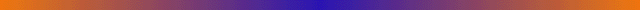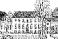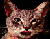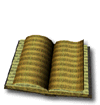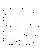predrag@nbi.dk